Geometric Progression(GP): Formulae based

Chapter 8 Class 11 Sequences and Series
Concept wise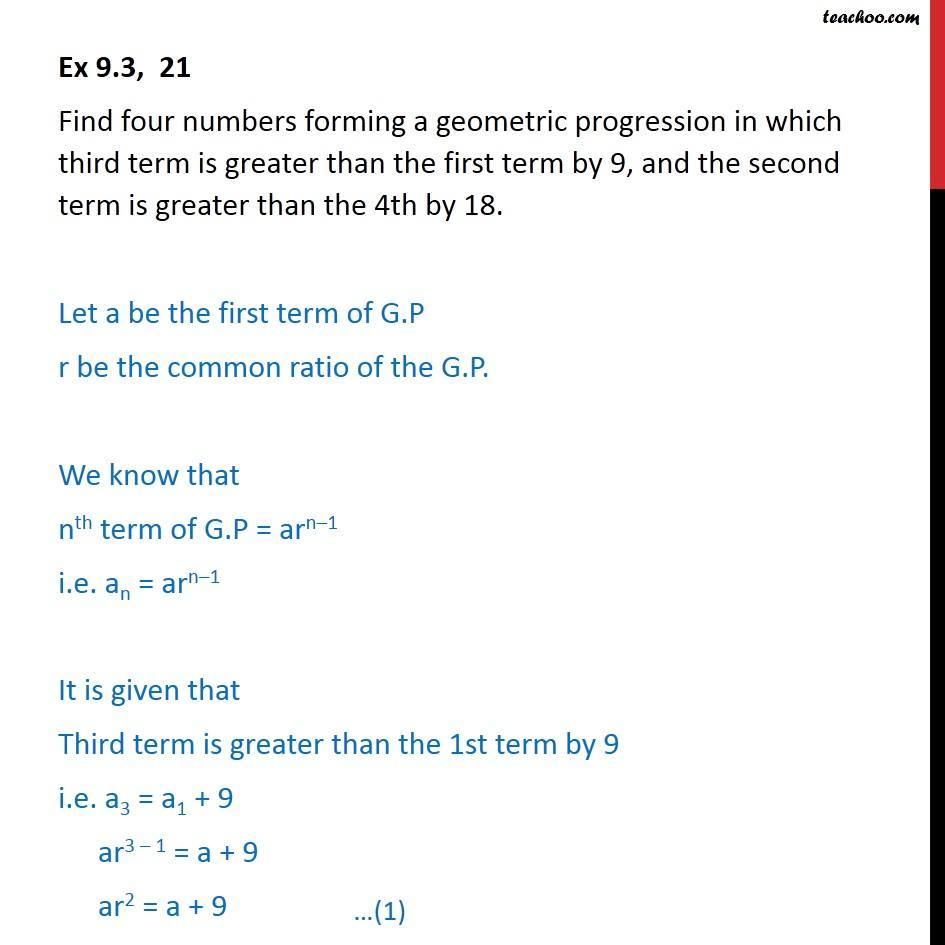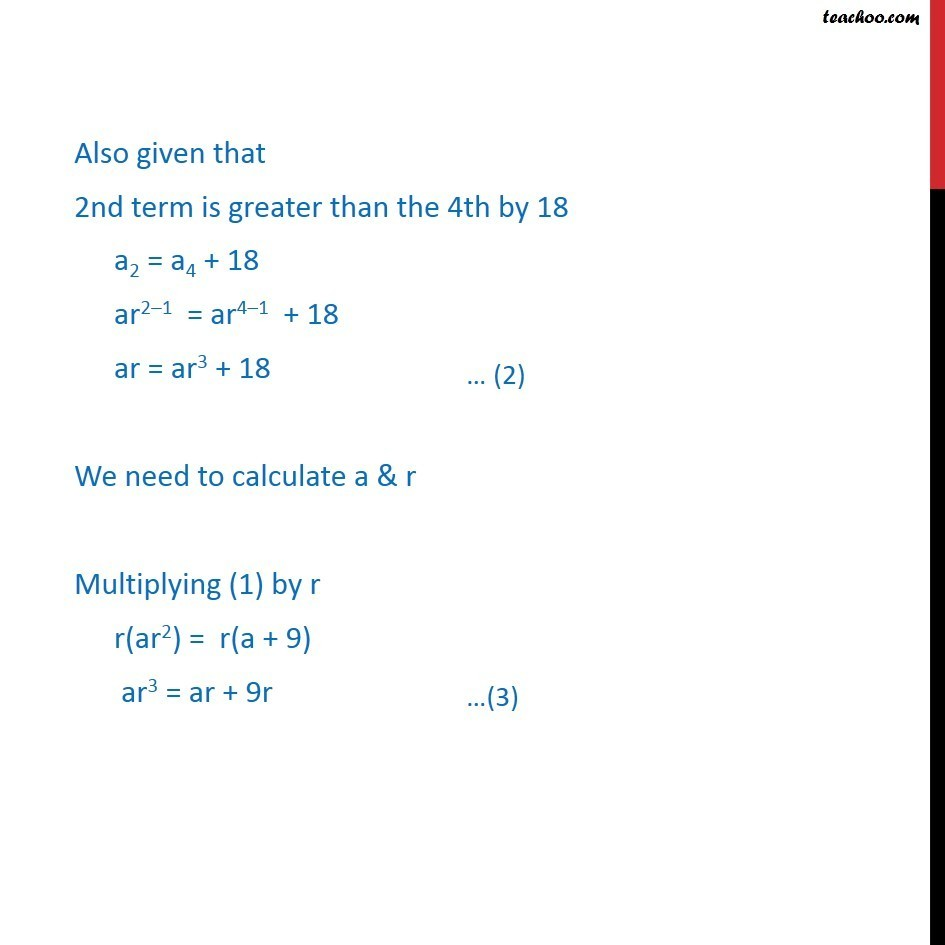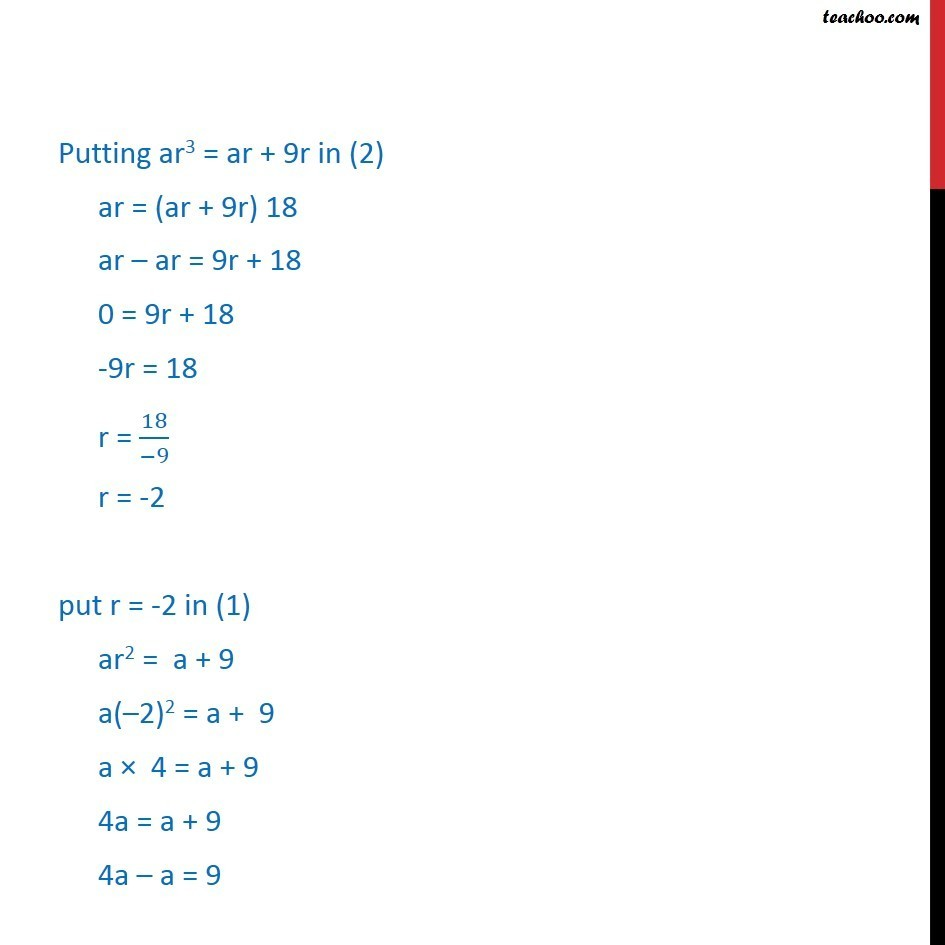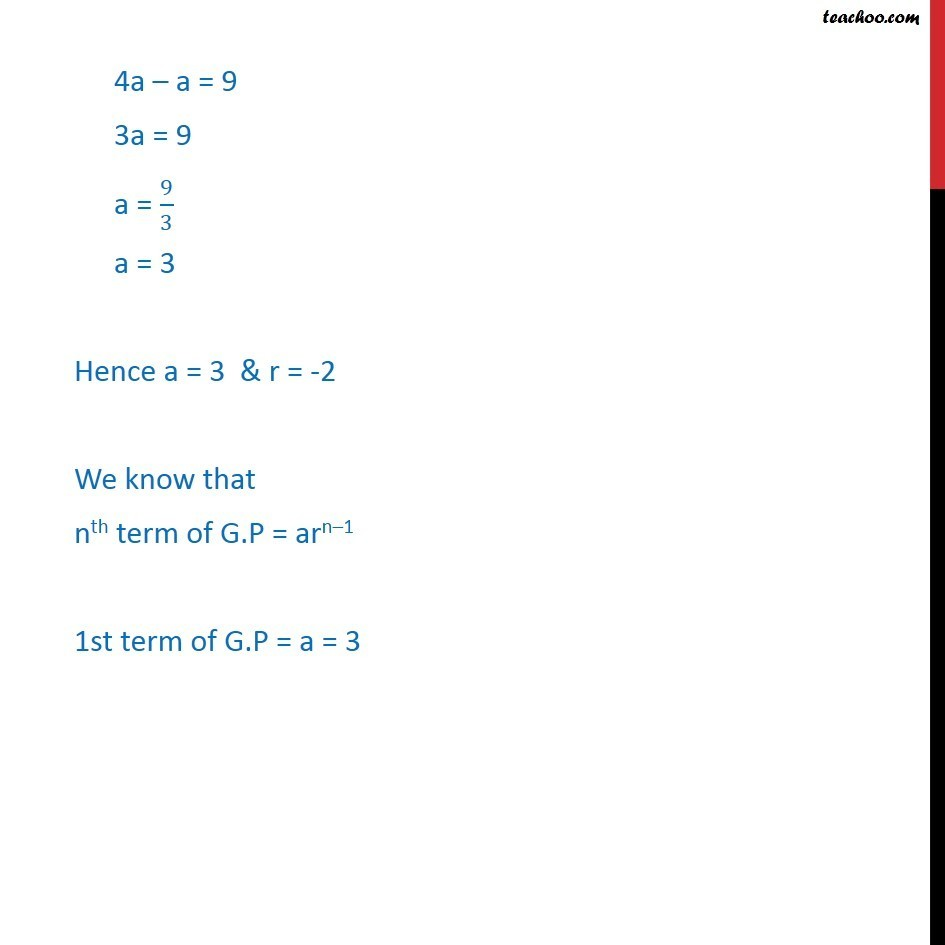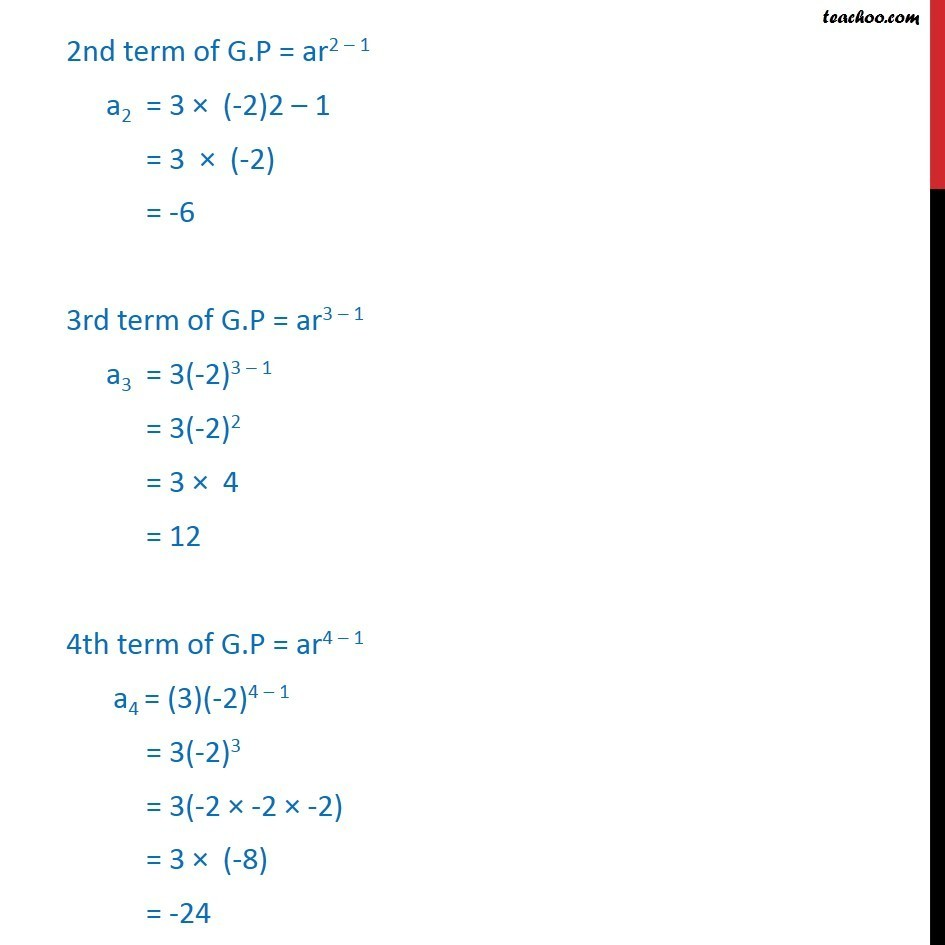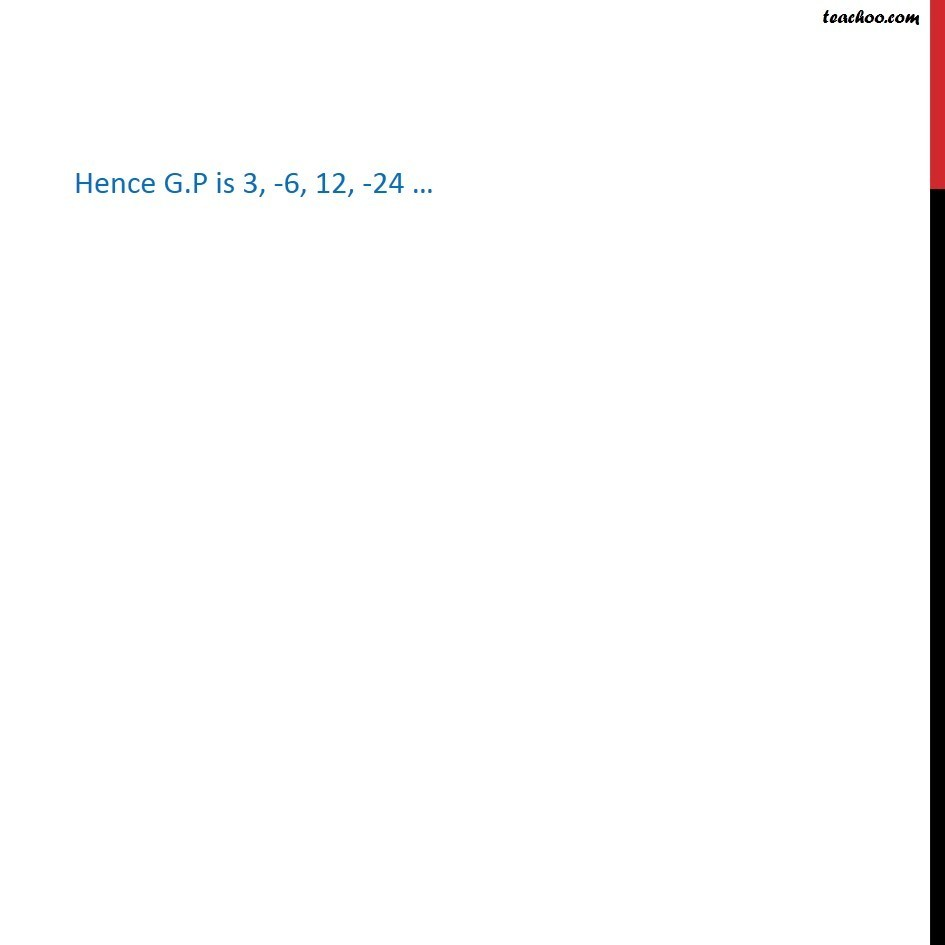Learn in your speed, with individual attention - Teachoo Maths 1-on-1 Class

### Transcript

Ex 8.2, 21 Find four numbers forming a geometric progression in which third term is greater than the first term by 9, and the second term is greater than the 4th by 18. Let a be the first term of G.P r be the common ratio of the G.P. We know that nth term of G.P = arn–1 i.e. an = arn–1 It is given that Third term is greater than the 1st term by 9 i.e. a3 = a1 + 9 ar3 – 1 = a + 9 ar2 = a + 9 Also given that 2nd term is greater than the 4th by 18 a2 = a4 + 18 ar2–1 = ar4–1 + 18 ar = ar3 + 18 We need to calculate a & r Multiplying (1) by r r(ar2) = r(a + 9) ar3 = ar + 9r Putting ar3 = ar + 9r in (2) ar = (ar + 9r) 18 ar – ar = 9r + 18 0 = 9r + 18 -9r = 18 r = 18/(−9) r = -2 put r = -2 in (1) ar2 = a + 9 a(–2)2 = a + 9 a × 4 = a + 9 4a = a + 9 4a – a = 9 4a – a = 9 3a = 9 a = 9/3 a = 3 Hence a = 3 & r = -2 We know that nth term of G.P = arn–1 1st term of G.P = a = 3 2nd term of G.P = ar2 – 1 a2 = 3 × (-2)2 – 1 = 3 × (-2) = -6 3rd term of G.P = ar3 – 1 a3 = 3(-2)3 – 1 = 3(-2)2 = 3 × 4 = 12 4th term of G.P = ar4 – 1 a4 = (3)(-2)4 – 1 = 3(-2)3 = 3(-2 × -2 × -2) = 3 × (-8) = -24 Hence G.P is 3, -6, 12, -24 …